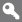X

to continue to ilmkidunya.com

X

to continue to ilmkidunya.com

X

### Register Type

Please Provide following information to Register

•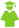Student
•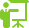Tutor
•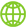Consultant
•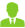Employer

# CSS Examination Syllabus - Applied Mathematics

Applied Mathematics ( Total Marks - 200 )

Paper I ( Marks - 100 )

Candidates will be asked to attempt any two questions from Section A and any three questions from Section B.

Section A

• Vector Analysis
Vector algebra, scalar and vector product of two or more vectors, Function of a scalar variable, Gradient, divergence and curl, Expansion formulae, curvilinear coordinates, Expansions for gradient, divergence and curl in orthogonal curvilinear coordinates, Line, surface and volume integrals, Green's, Stoke's and Gauss's theorms
• Statics
Composition and resolution of forces, Parallel forces, and couples, Equilibrium of a system of coplanar forces, Centre of mass and centre of gravity of a system of particles and rigid bodies, Friction, Principle of virtual work and its applications, equilibrium of forces in three dimensions.

Section B

• Dynamics
Tangential, normal, radial and transverse components of velocity and acceleration, Rectilinear motion with constant and variable acceleration, Simple harmonic motion, Work, Power and Energy, Conservative forces and principles of energy, Principles of linear and angular momentum, Motion of a projectile, Ranges on horizontal and inclined planes, Parabola of' safety. Motion under central forces, Apse and apsidal distances, Planetary orbits, Kepler's laws, Moments and products of inertia of particles and rigid bodies, Kinetic energy and angular momentum of a rigid body, Motion of rigid bodies, Compound pendulum, Impulsive motion, collision of two spheres and coefficient of restitution.

Paper II ( Marks - 100 )

Candidates will be asked to attempt any two questions from Section A. one question from Section B and two questions from Section C.

Section A

• Differential Equations
Linear differential equations with constant and variable coefficients, the power series method. Formation of partial differential equations. Types of integrals of partial differential equations. Partial differential equations of first order Partial differential equations with constant coefficients. Monge's method. Classification of partial differential equations of second order, Laplace's equation and its boundary value problems. Standard solutions of wave equation and equation of heat induction.

Section B

• Tensor
Definition of tensors as invariant quantities. Coordinate transformations. Contravariant and covariant laws of transformation of the components of tensors. Addition and multiplication of tensors, Contraction and inner product of tensors The Kronecker delta and Levi-Civita symbol. The metric tensor in Cartesian, polar and other coordinates, covariant derivatives and the Christoffel symbols. The gradient. divergence and curl operators in tensor notation.

Section C

• Elements of Numerical Analysis
Solution of non-linear equations, Use of x = g (x) form, Newton Raphson method, Solution of system of linear equations, Jacobi and Gauss Seidel Method, Numerical Integration, Trapezoidal and Simpson's rule. Regula falsi and interactive method for solving non-linear equation with convergence. Linear and Lagrange interpolation. Graphical solution of linear programming problems.

•
• Title
• Author
• 1
• Classical Mechanics
• Goldstein
• 2
• Lactures on Ordinary Differential Equations
• Hille, E.
• 3
• Lectures on Partial Differential Equations
• Petrovosky, I.G.
• 4
• Mechanics
• Symon, G.F.
• 5
• Mechanics
• Ghori, Q. K.
• 6
• Mathematical Pyysics, An Advanced Course
• Mikhin, S.G.
• 7
• Ordinary Differential Equations
• Easthan, M.S.P.
• 8
• Principles of Mechanics
• Synge and Griffith
• 9
• Principles of Mechanics
• Hauser
• 10
• Partial Differential Equations
• Sneddon, I.N.
• 11
• Theoratical Mechanics
• Beckker
• 12
• Theoratical Mechanics
• 13
• Theory of Ordinary Differential Equations
• Goddirgton, E.A. and N. Livenision
• 14
• Vector and Tensor Methods, Cartesian Tensors
• Charlton Jeffreya

## Check other Subjects and Syllabus below:

#### Compulsory Subjects

General Knowledge

### Optional Subjects:

(subjects carrying a total of 600 marks to be selected)

#### Group A

Subjects carrying not more than 200 marks can be opted.

#### Group B

Subject/s carrying not more than 200 marks can be opted.

#### Group C

Subjects carrying not more than 200 marks can be opted.

#### Group D (Science Subjects )

Subject/s carrying not more than 200 marks can be opted.

#### Group E (History Subjects )

Subjects carrying not more than 200 marks can be opted.

#### Group F

Subject/s carrying not more than 200 marks can be opted.
Note: International Law CANNOT BE opted in combination with International Relations.

#### Group G

Subjects carrying not more than 200 marks can be opted.

#### Group H (Regional Languages )

Subject/s carrying not more than 200 marks can be opted.
Regional Languages: Not more than one of the 4 subjects can be opted.

#### Group I (National and Foreign Languages )

Subjects carrying not more than 200 marks can be opted.
National/Foreign/Classical Languages: Not more than one of 4 subjects can be opted. Note: Urdu can not be combined with Regional Languages.